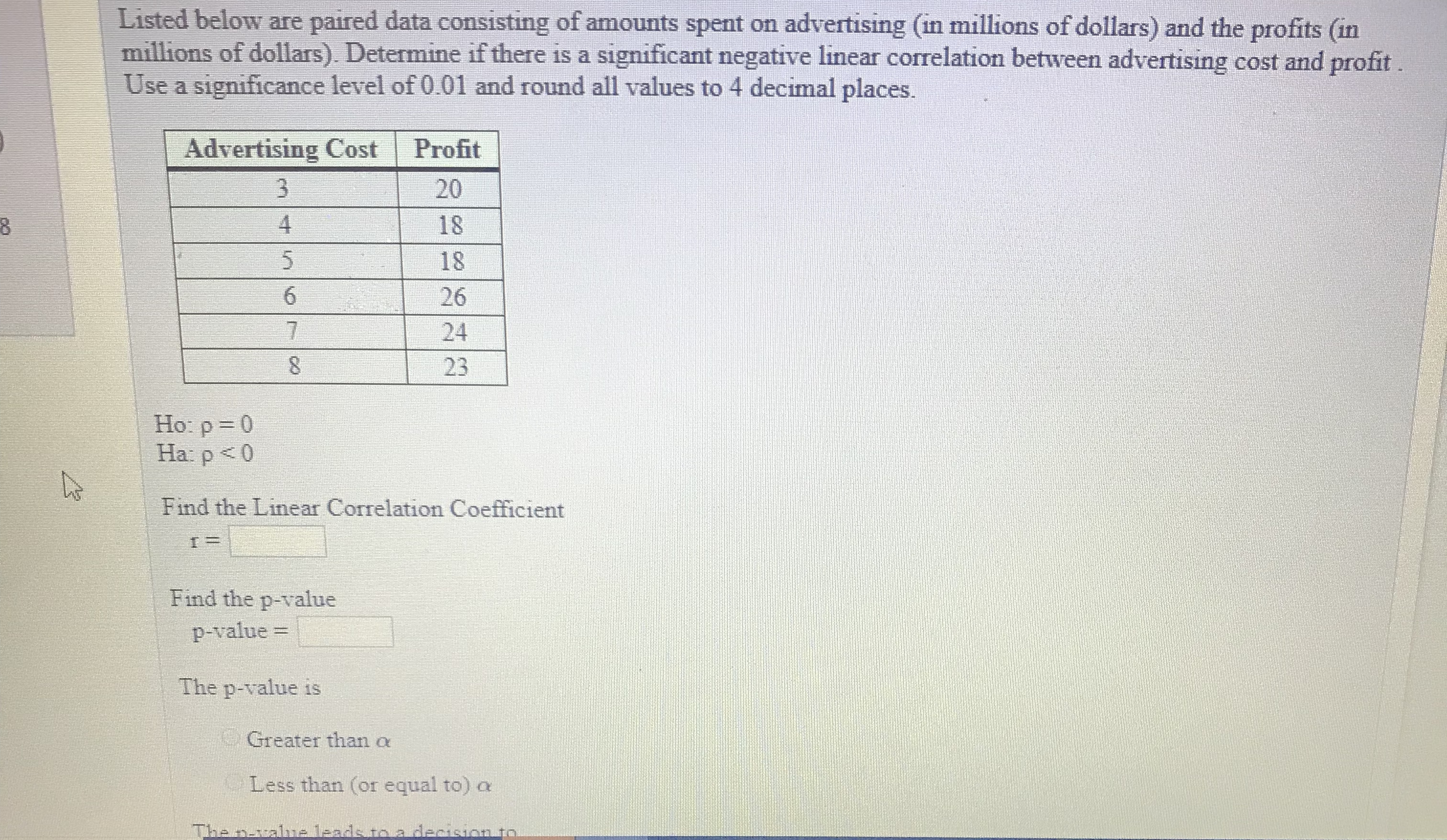# Listed below are paired data consisting of amounts spent on advertising (in millions of dollars) and the profits (inmillions of dollars). Determine if there is a significant negative linear correlation between advertising cost and profitUse a significance level of 0.01 and round all values to 4 decimal placesAdvertising Cost Profit2018182624234Ho: p-0Ha: p

Questionhelp_outlineImage TranscriptioncloseListed below are paired data consisting of amounts spent on advertising (in millions of dollars) and the profits (in millions of dollars). Determine if there is a significant negative linear correlation between advertising cost and profit Use a significance level of 0.01 and round all values to 4 decimal places Advertising Cost Profit 20 18 18 26 24 23 4 Ho: p-0 Ha: p<0 Find the Linear Correlation Coefficient Find the p-value p-value The p-value is Greater than α Less than (or equal to)a fullscreen
check_circleExpert Solution
Step 1

The correlation coefficient between two variables x and y can be calculated using the formula given below:

Step 2

Let x and y be the random variables that represent advertising cost and profit, both in million dollars respectively. Here, there are 6 data pairs of (x, y). So, n=6.

The computation of correlation coefficient is shown below:

Step 3

The provided alternative hypothesis suggests that the test is left-sided....

### Want to see the full answer?

See Solution

#### Want to see this answer and more?

Solutions are written by subject experts who are available 24/7. Questions are typically answered within 1 hour*

See Solution
*Response times may vary by subject and question
Tagged in

### Statistics